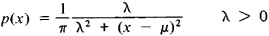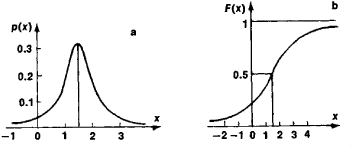# Cauchy Distribution

(redirected from Lorentzian)

## Cauchy distribution

[kō·shē dis·trə′byü·shən]
(statistics)
A distribution function having the form M /[π M 2+ (x-a)2], where x is the variable and M and a are constants. Also known as Cauchy frequency distribution.

## Cauchy Distribution

a special type of probability distribution of random variables. Introduced by Cauchy, it is marked by the densityThe characteristic function is

f(t) = exp (μit − λ ǀ t ǀ)

The Cauchy distribution is unimodal and symmetric with respect to the point x = μ, which is its mode and median. NoFigure 1. Cauchy distribution: (a) probability density, (b) distribution function

moments of positive order of a Cauchy distribution exist. Figure 1 depicts a Cauchy distribution for μ = 1.5 and λ = 1.

Mentioned in ?
References in periodicals archive ?
An important feature of the prepared two-point correlation is that the efective size of the structured supports of correlation at the M plane is fnite, and the biggest is the Lorentzian shaped support provided by the correlation cone associated to a single point source at the S-plane.
The approachwill be used also to establish new quantitative versions of classical geometric/functional inequalities for smooth riemannian manifolds and to make progress in long standing open problems for both riemannian and sub-riemannian manifolds.theme iii will investigate optimal transport in a lorentzian setting, where the ricci curvature plays a keyrole in einstein~s equations of general relativity.
Ingalahalli, G., Ricci solitons in Lorentzian -Sasakian manifolds, Acta Math.
A distorted signals are mathematically represented by Gaussian pulses, sech pulses, and Lorentzian pulses.
Minkowski space [E.sup.4.sub.1] corresponds to four dimensional Euclidean space with the induced Lorentzian metric defined as Equation 1:
In , a model similar to ours has been used to show that the early universe can undergo a spontaneous signature change from a Euclidean to Lorentzian phase.
In recent years, spacelike hypersurfaces in Lorentzian manifolds have been deeply studied not only from their mathematical interest, but also from their importance in general relativity.
The gain g falls off from its peak value at [[OMEGA].sub.B] by the typical Lorentzian lineshape:
Such CEST exchange peaks are commonly detected in a z-spectrum by performing an asymmetry analysis and presented as a magnetization transfer asymmetry ratio ([MTR.sub.asym]) or by fitting analysis using some mathematical functions like Gaussian and/or Lorentzian curve fitting methods.
Additionally, to improve the experimental data fitting, the spectrum in the regions around the LO and 2LO peaks was deconvoluted through several additional Lorentzian functions using the Fityk software[TM] .
Herein, it is worth mentioning that the shape of peak can be described as a combination of two functions: Gaussian associated with microstrain and Lorentzian associated with crystallite size (Figures 1, 2, and 3) [20, 21].
Now consider an observer in local Minskowskian system with radial velocity [omega], in the Lorentzian system we can write:

Site: Follow: Share:
Open / Close